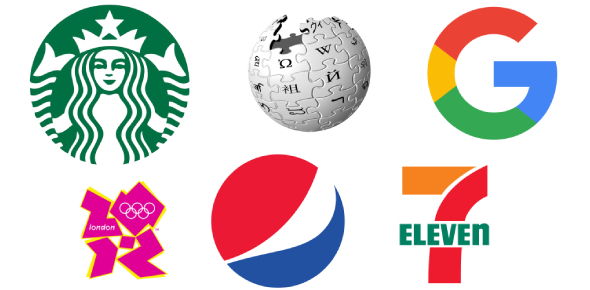# Metals & Their Reactions

8 Questions | Attempts: 1798
ShareSettings• 1.
Complete the word equation: Zinc + hydrochloric acid → ?
• A.

Zinc hydrochloric + acid

• B.

Zinc chloride + hydrogen

• C.

Zinc chloric + hydrogen

• D.

Zinc hydroacid + chloric

• 2.
Which of the following elements is a good conductor used in electrical wiring?
• A.

Iron

• B.

Sulfur

• C.

Copper

• D.

Carbon

• 3.
Which two chemicals can be used to make copper nitrate?
• A.

Copper hydroxide and nitric acid

• B.

Copper oxide and hydrochloric acid

• C.

Copper and nitrogen dioxide

• D.

Copper carbonate and sulfuric acid

• 4.
Complete the word equation: Magnesium + nitric acid ?
• A.

Magnesium nitric + acid

• B.

Magnesium nitrate + oxygen

• C.

Magnesium nitrate + carbon dioxide + water

• D.

Magnesium nitrate + hydrogen

• 5.
Which of the following metals reacts most vigorously with cold water?
• A.

Sodium

• B.

Iron

• C.

Copper

• D.

Gold

• 6.
Which of the following metals could produce zinc metal, if heated with zinc oxide?
• A.

Aluminium

• B.

Magnesium

• C.

Copper

• D.

Silver

• 7.
Which of the following metal mixtures could be separated from one another by adding dilute hydrochloric acid? Assume that the metals simply mixed, and have not formed an alloy.
• A.

Sodium and potassium

• B.

Magnesium and zinc

• C.

Magnesium and copper

• D.

Copper and silver

• 8.
Complete the word equation: Zinc carbonate + hydrochloric acid ?
• A.

Zinc chloride + carbon dioxide

• B.

Zinc chloride + carbon dioxide + water

• C.

Zinc chloride + water

• D.

Zinc chloride + oxygen

## Related TopicsBack to top
×

Wait!
Here's an interesting quiz for you.